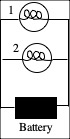# Directions:  Click here to download the Prediction Sheet where you will enter your predictions and answers. Write your name at the top to record your presence and participation in these demonstrations.  For each demonstration below, write your prediction on this sheet before making any observations. You may be asked to send this sheet to your instructor.

 Demonstration 1: A resistor (a device that obeys Ohm’s law) is connected to a variable source of voltage. Sketch on the right your prediction of a graph of the current that flows through the resistor as the voltage across the resistor is increased starting from zero. After you have made your prediction, open the simulation: https://phet.colorado.edu/sims/html/circuit-construction-kit-dc/latest/circuit-construction-kit-dc_en.html Click on Lab and construct the circuit. You can look at a picture of the correct circuit here. Adjust the battery voltage in equal 5 volt steps by clicking once on the battery and adjusting the slide switch. Record the values in the table and sketch the graph with a dashed line. See how the current through the bulb varies and compare it to your prediction. Explain. See how the current through the resistor varies and compare it to your prediction. Explain.     How would you calculate the resistance of the resistor by reading values from the graph?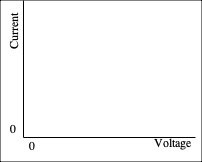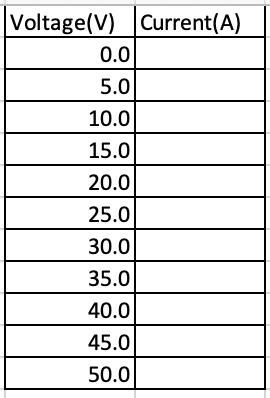Demonstration 2: The resistance of most conductors increases as the temperature increases. As more current flows through the filament of a light bulb, the temperature of the filament gets higher. A light bulb is connected to a variable source of voltage. Sketch on the right your prediction of a graph of the current that flows through the light bulb as the voltage across the light bulb is increased starting from zero. After you have made your prediction, again use the simulation: https://phet.colorado.edu/sims/html/circuit-construction-kit-dc/latest/circuit-construction-kit-dc_en.html Click on Lab and construct the circuit. You can look at a picture of the correct circuit here. Adjust the battery voltage in equal 5 volt steps by clicking once on the battery and adjusting the slide switch, only this time, increase the resistance of the bulb by 0.5 ohm for each voltage step. Record the values in the table and sketch the graph with a dashed line. See how the current through the bulb varies and compare it to your prediction. Explain.   Does a light bulb obey Ohm’s law?   How would you find the resistance of the light bulb at any particular voltage?Demonstration 3: Two light bulbs are connected with the same potential difference (voltage) across their terminals. Bulb 2 has twice the resistance of Bulb 1. Predict which bulb has a larger current flowing through it, or do they both have the same current? Again use the simulation https://phet.colorado.edu/sims/html/circuit-construction-kit-dc/latest/circuit-construction-kit-dc_en.html Construct the same circuit as for Demonstration 2. Find the current for the two bulbs with the same applied voltage. Compare these values to your prediction and explain.       Recall that the power delivered to a device is P = IV. Predict which bulb has the larger power delivered to it, or do they both have the same power? Which bulb is brighter, or are they both just as bright?   Use the same simulation to explore your predictions. Compare and explain. Demonstration 4: Seven bulbs are connected to a battery (B) as shown on the right. Define series connection, and list all combinations of bulbs that are connected in series. After you have made your prediction, check the answer here. Was  your prediction correct? Why or why not?   Define parallel connection, and list all combinations of bulbs that are connected in parallel. After you have made your prediction, check the answer here. Was  your prediction correct? Why or why not?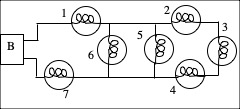Demonstration 5: Two different bulbs are connected to a battery as shown on the right. Bulb 1 has a smaller resistance than Bulb 2. Predict the current through Bulb 1 as compared to the current through Bulb 2. After you have made your porediction, use the same simulation https://phet.colorado.edu/sims/html/circuit-construction-kit-dc/latest/circuit-construction-kit-dc_en.html Construct the circuit with two ammeters to measure the currents through Bulb 1 and Bulb 2. You can look at a picture of the correct circuit here. Adjust the battery voltage to some non-zero value and observe the currents through the two bulbs. Compare to your prediction and explain.   Now predict the voltage across Bulb 1 as compared to the voltage across Bulb 2. After you have made your porediction, use the same simulation, removing the ammeters and adding a voltmeter first across Bulb 1 and then across Bulb 2. You can look at a picture of the correct circuit with the voltmeter across Bulb 1 here. Adjust the battery voltage to some non-zero value and observe the voltages across the two bulbs. Compare to your prediction and explain.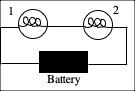Demonstration 6: Two different bulbs are connected to a battery as shown on the right. Bulb 1 has a smaller resistance than Bulb 2. Predict the current through Bulb 1 as compared to the current through Bulb 2. After you have made your porediction, use the same simulation https://phet.colorado.edu/sims/html/circuit-construction-kit-dc/latest/circuit-construction-kit-dc_en.html Construct the circuit with two ammeters to measure the currents through Bulb 1 and Bulb 2. You can look at a picture of the correct circuit here. Adjust the battery voltage to some non-zero value and observe the currents through the two bulbs. Compare to your prediction and explain.   Now predict the voltage across Bulb 1 as compared to the voltage across Bulb 2, and also compared to the voltage of the batgtery. After you have made your porediction, use the same simulation, removing the ammeters and adding a voltmeter first across Bulb 1, then across Bulb 2 and finally across the battery. You can look at a picture of the correct circuit with the voltmeter across Bulb 1 here. Adjust the battery voltage to some non-zero value and observe the voltages across the two bulbs, and across the battery. Compare to your prediction and explain.